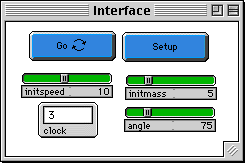GASLAB:  SINGLE COLLISION

WHAT IS IT?
-----------
This program simulates the behavior of gas molecules. It is
related to one of the original CM StarLogo applications (under
the name GPCEE) and is now ported to Macintosh StarLogoT as part
of the Connected Mathematics Modeling Project.

This is one in a series of GasLab models that use the same basic
rules for what happens when molecules run into each other.
Each one has different features in order to show different
aspects of the behavior of gases.  This model is simplified to
show the collision of only two molecules, since this event is so
hard to watch when there are many molecules on the screen.
This basic collision is the same as what is used in all the
other models.

Molecules are modeled as perfectly elastic particles with no
internal energy except that which is due to their motion.
Collisions between molecules are elastic.

The exact way two molecules collide is as follows:
1.  Two turtles "collide" if they find themselves on the same
patch.  In this model, two turtles are aimed toward each other
with the same speed so that they will collide at the origin.
2.  An axis is chosen, as if they were two balls that hit,
and this axis were the line connecting their centers.  This
angle, called theta, can range between 0 and 360 degrees, and is
set by the angle slider.  Note that it is measured clockwise
from straight up (the positive y-axis).
3.  The turtles exchange momentum and energy only along that
axis, according to the conservation of momentum and energy.
This calculation is done in the center-of-mass system along
that axis.
4.  The new velocity of each turtle is translated back from the
center-of-mass system to the screen reference frame.
5. Each turtle is assigned its new velocity, energy, and heading.

HOW TO USE IT
-------------

The initial speed and mass of each turtle is set with sliders.
Each one traces its path with yellow-stamped patches that
gradually fade away.  The center of mass of the pair of turtles
is traced in white.   The angle at which they strike each other
(as if they were billiard balls) is set with the "angle"
slider.

This model is initially set to run the simplest possible

If the model is left to run, the two turtles are automatically
set back to their initial positions and velocities after each
collision, and the angle (theta) advances 15 degrees, up to 360.
The angle can also be changed by moving the angle slider with
the pointer.

RUNNING the MODEL
-----------------

Set the angle (theta) to zero.  Draw a picture representing the
two balls as they collide, with their two faces touching.  Make
the line connecting their centers be the same as theta. Draw
vectors representing their motion.

Push Setup and then Go.  You can "freeze" the run at any time
by pushing the Go button again. Note the paths of the two
particles.  Can you make sense of what they do?

Cycle through all the values of theta.  Compare each situation

If you want to run the collision very slowly, run "go"
repeatedly in the Command Center.  Each "go" advances the
particles one tick only.

THINGS TO NOTICE
----------------

The first collision (theta = 0) looks as if the two particles
miss each other.  What is happening?

At theta=45 degrees, the particles go off at right angles.
Why?  Draw a picture of what is happening at the moment of
collision.

At theta=90 degrees, the two particles reverse direction.
Why?

What is the motion of the center of mass?  What would you
expect it to be?

THINGS TO TRY
-------------

Change the initial positions and headings of the two particles.
Do this under the Setup procedure.  The (who = 0) turtle is gray
and the (who =1) turtle is red.  As a simple case, set one on
the y-axis and the other on the x-axis, each one heading toward
the origin.  The center of mass is no longer stationary. Note
its path.  Is it what you would expect?

If the center of mass is not stationary, the two particles
often have different speeds after they collide, even though
they have identical initial speeds and masses!  Why does this
happen?  How can this satisfy the conservation of both energy
and momentum?

The fact that the velocities are not always the same after
every kind of collision is essential to getting a distribution
of velocities among identical particles after many collisions,
which is what we observe with molecules in a gas.

Does this seem like a reasonable model for colliding molecules?
When is it reasonably valid, and when is it decidely NOT valid?

Should theta be picked randomly -- each theta has an equal
probability -- or in some other way?  Would this change the
eventual velocity distribution among many particles?

After you have gotten used to observing and understanding these
simple collisions, go to the GAS IN A BOX model.  Especially
watchthe particle whose path is traced in yellow.  Does it make
sense?  Can you picture each collision?

EXTENDING THE MODEL
-------------------

Have the masses of the two particles be different.

Have the initial speeds of the two particles be different.

Record the velocities of each particle after each collision.
After you have several sets of velocities, look at the entire
velocity distribution. What do you notice? Is it the Maxwell-
Boltzman distribution?

STARLOGO FEATURES
-----------------
Note that angles are measured clockwise from STRAIGHT UP,that
is, along the positive y-axis.  This is not the standard
convention.  The "a-atan" function in the code changes the sign